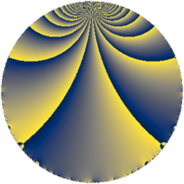# Properties

 Label 65.2.lLevel $65$ Weight $2$ Character orbit 65.l Rep. character $\chi_{65}(4,\cdot)$ Character field $\Q(\zeta_{6})$ Dimension $8$ Newform subspaces $1$ Sturm bound $14$ Trace bound $0$

# Related objects

## Defining parameters

 Level: $$N$$ $$=$$ $$65 = 5 \cdot 13$$ Weight: $$k$$ $$=$$ $$2$$ Character orbit: $$[\chi]$$ $$=$$ 65.l (of order $$6$$ and degree $$2$$) Character conductor: $$\operatorname{cond}(\chi)$$ $$=$$ $$65$$ Character field: $$\Q(\zeta_{6})$$ Newform subspaces: $$1$$ Sturm bound: $$14$$ Trace bound: $$0$$

## Dimensions

The following table gives the dimensions of various subspaces of $$M_{2}(65, [\chi])$$.

Total New Old
Modular forms 16 16 0
Cusp forms 8 8 0
Eisenstein series 8 8 0

## Trace form

 $$8 q - 2 q^{4} - 6 q^{6} - 8 q^{9} + O(q^{10})$$ $$8 q - 2 q^{4} - 6 q^{6} - 8 q^{9} - 4 q^{10} + 12 q^{14} - 6 q^{15} - 2 q^{16} - 12 q^{19} + 24 q^{20} + 12 q^{24} - 18 q^{26} - 10 q^{30} + 6 q^{35} - 4 q^{36} + 20 q^{39} - 12 q^{40} + 12 q^{45} + 42 q^{46} + 16 q^{49} - 12 q^{50} + 30 q^{54} - 14 q^{55} - 12 q^{56} - 60 q^{59} + 24 q^{61} - 64 q^{64} - 24 q^{65} - 28 q^{66} + 12 q^{71} - 42 q^{74} - 8 q^{75} + 6 q^{76} + 48 q^{79} + 18 q^{80} - 4 q^{81} - 6 q^{84} + 42 q^{85} + 48 q^{89} + 16 q^{90} - 12 q^{91} + 40 q^{94} - 6 q^{95} + O(q^{100})$$

## Decomposition of $$S_{2}^{\mathrm{new}}(65, [\chi])$$ into newform subspaces

Label Dim $A$ Field CM Traces $q$-expansion
$a_{2}$ $a_{3}$ $a_{5}$ $a_{7}$
65.2.l.a $8$ $0.519$ 8.0.49787136.1 None $$0$$ $$0$$ $$0$$ $$0$$ $$q+(\beta _{1}+\beta _{5})q^{2}+(\beta _{3}+\beta _{5})q^{3}+(\beta _{2}+\beta _{4}+\cdots)q^{4}+\cdots$$